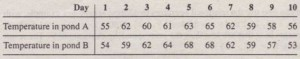Analysis of Temperature Data

The arrays temp_A and temp_B given in the table contain the water temperature in degrees Fahrenheit of two ponds measured at noon for JO days. Determine how many days the temperature of pond A was above 60′. On what days did this occur? Determine the temperature of pond A on the days when it was greater than or equal to the temperature of pond B.Solution

The temperature of pond A was above 60° on five days: days 2, 4, 5, 6, and 7. The temperature of pond A was above the temperature of pond B or six days. On those days its temperature was 55, 62, 62, 59, 58, and 56° respectively